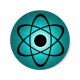# Scientific notation

Scientific notation

09/01/16

“How do scientists and engineers represent complex numbers?”

When working in a technical subject, you will probably have to deal with numbers that are either extremely large or extremely small, or have to work with empirically obtained values. For example, the number 602000000000000000000000 is really to read, as well as 0.000027. So how can we make them simpler and more accurate?

Well, let’s try to tackle this problem ourselves. What if we took our understanding of significant figures, and applied it to this problem? Since the only numbers that  we actually need to care about is significant figures, how about we just remove all of the unnecessary zeroes? And how can we accomplish this? Well since we know that if we multiply or divide anything by 10 we will just shift the zeroes behind the numbers, how about we simplify all of the extra zeroes into a power of ten? the For example, when we have the number 602000000000000000000000, we can turn this in to 6.02*10^23. And for the number 0.000027, we can shift it into 2.7*10^-5. This way of working so much simpler! Scientists and Engineers have termed this framework scientific notation.

Now how can we apply this system for calculations? Well, first let’s divided it into two cases, multiplication/division, and addition/subtraction.  In the first case, we will multiply both numbers and round our final answer to the number of significant figures of the variable with the lowest amount of sig-figs. For example, 5.2*10.81 will be 56 instead of 56.212 since the former only has two significant figures. For addition and subtraction, We will simply put all of the numbers in terms of the highest amount of digits after the decimal place, and then round to the lowest amount of significant figures. Let’s do an example. Suppose we have the numbers 3.14 and 2.1, and 1. When we ad the numbers together, we will notice that 3.14 has the highest amount of digits (2), and then rewrite everything else accordingly to become 3.14 + 2.10 +1.00 = 6.24, and then we will round down to one significant figure to finally arrive at 6.

Scientific notation is an amazing tool for scientific accuracy, because when working with complicated systems such as mechanical engineering precision or particle physics, a single bit of inaccuracy could destroy all of our hard work!

## 2 thoughts on “Scientific notation”

1.Vincent Phang says:

Firstly, 0.000027 = 2.7*10^-5
NOT 2.7*10^-4

Second, in scientific usage, we go for accuracy. A single digits of can throw the entire procedure off, such as in astronomy or astrophysics. Remember it is a very big universe out there. In navigation, a digit off can throw a course off by miles/kilometers. So rounding off 56.212 is unacceptable. The other technical ( mathematical) reason for not rounding off is that both numbers are REAL numbers. So, we must return REAL numbers in our answers unless the problem stipulates to return an WHOLE ( INTEGER) number as the answer. Same applies to 8.31+1.4. To maintain the integrity off the numbers, the answer is correctly 9.71. It should not be cut off as 9.7. What of the system is 8.36+1.4? Then clearly, truncating the answer too 9.7 is highly inadequate! Case in point, of the computer were built to truncate the numbers in the fashion mentioned, what cartography would result if there is a series of numbers to add up, such as 8.31+1.4+6.24+0.99=16.94. Would the correct answer be 16.9? 16.8? This alone shows the folly of truncating numbers. A better solution is to rework the problem as 8.31+1.40 so that a more complete picture may be made available. This will also make available several options, one of which is to minimize confusion. It also helps the learning, and thus understanding, process. Compare the problems presented below

8.31
1.4
6.24
0..99 (+)

Compare to

8.31
1.4
6.24
0.99 (+)

Would either above problem bee better represented (rewritten) as

8.31
1.40
6.24
0.99 (+)

Like

1.Isaac Gendler says:

Thank you very much for your feedback, your points raised have now been implemented in the latest revision. I hope you enjoy, and make any criticism you would like for future posts.

Like